Custom SearchPOSITIVE INTEGERS CHAPTER 2 The purpose of this chapter is to review the methods of combining integers. We have already used one combination process in our discussion of counting. We will extend the idea of counting, which is nothing more than simple addition, to develop a systematic method for adding numbers of any size, We will also learn the meaning of subtraction, multiplication, and division. ADDITION AND SUBTRACTION In the following discussion, it is assumed that the reader knows the basic addition and subtraction tables, which present such facts as the following: 2 + 3 = 5,9 + 8 = 17, 8 - 3 = 5, etc. The operation of addition is indicated by a plus sign (+) as in 8 + 4 = 12. The numbers 8 and 4 are ADDENDS and the answer (12) is their SUM. The operation of subtraction is indicated by a minus sign (-) as in 9 - 3 = 6. The number 9 is the MINUEND, 3 is the SUBTRAHEND, and the answer (6) is their DIFFERENCE. REGROUPING Addition may be performed with the addends arranged horizontally, if they are small enough and not too numerous. However, the most common method of arranging the addends is to place them in vertical columns. In this arrangement, the units digits of all the addends are a lined vertically, as are the tens digits, the hundreds digits, etc. The following example shows three addends arranged properly for addition: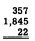It is customary to draw a line below the last addend, placing the answer below this line. Subtraction problems are arranged in columns in the same manner as for addition, with a line at the bottom and the answer below this line. Carry and Borrow Problems involving several addends, with two or more digits each, usually produce sums in one or more of the columns which are greater than 9. For example, suppose that we perform the following addition: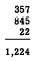The answer was found by a process called "carrying." In this process extra digits, generated when a column sum exceeds 9, are carried to the next column to the left and treated as addends in that column. Carrying may be explained by grouping the original addends. For example, 357 actually means 3 hundreds plus 5 tens plus 7 units. Rewriting the problem with each addend grouped in terms of units, tens, etc., we would have the following: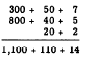The "extra" digit in the units column of the answer represents 1 ten. We regroup the columns of the answer so that the units column has no digits representing tens, the tens column has no digits representing hundreds, etc., as follows: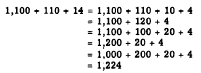When we carry the 10 from the expression 10 + 4 to the tens column and place it with the 110 to make 120, the result is the same as if we had added 1 to the digits 5, 4, and 2 in the tens column of the original problem. Therefore, the thought process in addition is as follows: Add the 7, 5, and 2 in the units column, getting a sum of 14. Write down the 4 in the units column of the answer and carry the 1 to the tens column. Mentally add the 1 along with the other digits in the tens column, getting a sum of 12. Write down the 2 in the tens column of the answer and carry the 1 to the hundreds column. Mentally add the 1 along with the other digits in the hundreds column, getting a sum of 12. Write down the 2 in the hundreds column of the answer and carry the 1 to the thousands column. If there were other digits in the thou- sands column to which the 1 could be added, the process would continue as before. Since there are no digits in the thousands column of the original problem, this final 1 is not added to anything, but is simply written in the thousands place in the answer. The borrow process is the reverse of carrying and is used in subtraction. Borrowing is not necessary in such problems as 46 - 5 and 58 - 53. In the first problem, the thought process may be "5 from 6 is 1 and bring down the 4 to get the difference, 41." In the second problem, the thought process is "3 from 8 is 5" and "5 from 5 is zero," and the answer is 5. More explicitly, the subtraction process in these examples is as follows: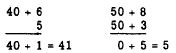This illustrates that we are subtracting units from units and tens from tens. Now consider the following problem where borrowing is involved: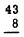If the student uses the borrowing method, he may think "8 from 13 is 5 and bring down 3 to get the difference, 35." In this case what actually was done is as follows: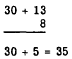A 10 has been borrowed from the tens column and combined with the 3 in the units column to make a number large enough for subtraction of the 8. Notice that borrowing to increase the value of the digit in the units column reduces the value of the digit in the tens column by 1. Sometimes it is necessary to borrow in more than one column. For example, suppose that we wish to subtract 2,345 from 5,234. Grouping the minuend and subtrahend in units, tens, hundreds, etc., we have the following:Borrowing a 10 from the 30 in the tens column, we regroup as follows: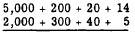The units column is now ready for subtraction. By borrowing from the hundreds column, we can regroup so that subtraction is possible in the tens column, as follows: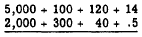In the final regrouping, we borrow from the thousands column to make subtraction possible in the hundreds column, with the following result: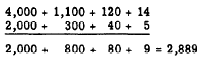In actual practice, the borrowing and regrouping are done mentally. The numbers are written in the normal manner, as follows: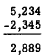The following thought process is used: Borrow from the tens column, making the 4 become 14. Subtracting in the units column, 5 from 14 is 9. In the tens column, we now have a 2 in the minuend as a result of the firs-t borrowing operation. Some students find it helpful at first to cancel any digits that are reduced as a result of borrowing, jotting down the digit of next lower value just above the canceled digit. This has been done in the following example: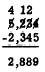After canceling the 3, we proceed with the subtraction, one column at a time. We borrow from the hundreds column to change the 2 that we now have in the tens column into 12. Subtracting in the tens column, 4 from 12 is 8. Proceeding in the same way for the hundreds column, 3 from 11 is 8. Finally, in the thousands column, 2 from 4 is 2.  Practice problems. In problems 1 through 4, add the indicated numbers. In problems 5 through 8, subtract the lower number from the upper.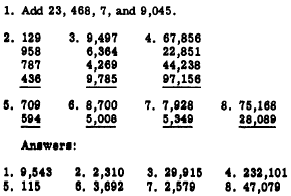Numbers that have a unit of measure associated with them, such as yard, kilowatt, pound, pint, etc., are called DENOMINATE NUMBERS. The word ‘denominate" means the numbers have been given a name; they are not just abstract symbols , To add denominate numbers, add all units of the same kind. Simplify the result, if possible. The following example illustrates the addition of 6 it 8 in. to 4 ft 5 in:Since 13 in. 113 the equivalent of 1 ft 1 in., we regroup the answer as 11 it 1 in. A similar problem would be to add 20 degrees 44 minutes 6 seconds to 13 degrees 22 minutes 5 seconds. This is illustrated as follows:This answer is regrouped as 34 deg 6 min 11 sec. Numbers must be expressed fn units of the same kind, in order to be combined. For instance, (he sum of 6 kilowatts plus 1 watt is not 7 kilowatts nor is it 7 watts. The sum can only be indicated (rather than performing the operation) unless some method is used to write these numbers in units of the same value. Subtraction of denominate numbers also involves the regrouping idea. If we wish to subtract 16 deg 8 min 2 set from 28 deg 4 min 3 set, for example, we would have the following arrangement: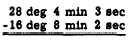In order to subtract 8 min from 4 min we regroup are follows: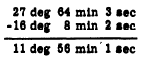Practice problems. In problems 1,2, and 3 add. In problems 4, 5, and 6 subtract the lower number from the upper.Answers: 1. 9 yd 2 ft 2 in. 2. 18 hr 39 min 37 set 3. 36 wks 6 days 5 hr 4. 8 hr 34 min 35 set 5. 77 deg 50 min 46 set  6. 12 wks 2 days 19 hr